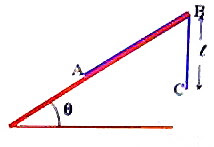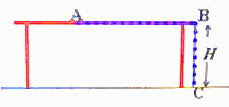## Pages

`“Life is like riding a bicycle.  To keep your balance you must keep moving.”–Albert Einstein`

## Sunday, October 26, 2008

### AP Physics B & C- Answers to Free Response Practice Questions on Newton’s Laws

In the post dated 24th October 2008 two free response practice questions on Newton’s laws were given. As promised, I give below model answers along with the questions:(1) Fig. 1 shows a small block of mass M1 placed on the rough inclined surface of a large wedge shaped block of mass M2 which is at rest on a rough horizontal table top. The coefficients of static friction and kinetic friction (between M1 and M2) are respectively μs and μk. Now answer the following questions (a), (b) and (c):

(a) Block M1 is just going to slide down. Draw the free body diagram for block M1 showing and identifying all the forces acting on it. (Represent M1 by a dot).

(b) What is the magnitude of the frictional force on M1 if it is at rest and does not show any sign of sliding down? Justify your answer.

(c) What is the magnitude of the frictional force on M1 if the mass M1 is sliding down? Justify your answer.Now suppose that there is no friction between the blocks M1 and M2 as well as between M2 and the table top. The system of masses M1 and M2 is connected to another mass M3 by a light inextensible string passing over a frictionless pulley of negligible mass as shown in fig.2. The mass M3 is such that the block M1 is prevented from sliding down along the block M2. Now answer the following questions (d) and (e):

(d) Determine the common acceleration of M1 and M2.

(e) Show that the condition for the block M1 not sliding along the block M2 is

M3 = (M1+M2)/(cot θ – 1)(a) The free body diagram is shown in the adjoining figure. The forces on the block are:

(i) Weight M1g of block M1

(ii) Normal force N1 (exerted by the inclined surface) which is equal to M1g cosθ.

(iii) Force of limiting static friction (Ff) which is equal to μsM1g cosθ where μs is the coefficient of static friction.

(b) When the block is at rest on the inclined surface, the frictional force is equal and opposite to the component M1g sinθ of the weight of the block along the plane. So the magnitude is M1g sinθ.

(c) The frictional force on M1 when it is sliding down is μkM1g cosθ since the coefficient of kinetic friction μk is to be used in this case.

(d) The force driving the system is the weight M3g of the mass M3. The total mass moved is (M1 + M2 + M3) so that the common acceleration a is given by

a = M3g/(M1 + M2 + M3)

(e) If M1 is not to slide along the block M2, the component of the above common acceleration along the incline must be equal to the relative acceleration of M1 (with respect to M2) along the incline: Thus

a cosθ = g sinθ

Substituting for a, we have

M3g cosθ /(M1 + M2 + M3) = g sinθ

Therefore, M3 cosθ/sinθ = M1 + M2 + M3 so that

M3 = (M1+M2)/(cot θ – 1)(2) The adjoining figure shows a uniform chain of length L placed on a rough inclined plane of angle θ with a portion of length (of the chain) overhanging from the top edge B of the incline. The coefficient of friction between the chain and the inclined plane is μ. Now answer the following questions (a), (b) and (c):

(a) In the condition shown in the figure, the chain is just going to slide up the incline. Obtain an expression for the overhanging length of the chain in terms of the given parameters.

(b) If the chain were just going to slide down the incline, will the value of the overhanging length of the chain be different from the value obtained in part (a)?

Put a tick (√) mark against the correct option among (i), (ii) and (iii) given below:

(i) Yes_____ (ii) No_____ (iii) The answer will depend on the angle of the plane.

(c) If there is no friction between the chain and the inclined plane, determine the value of the overhanging length of the chain that will make it just start sliding down the plane.The chain is now placed on a frictionless horizontal table of height H so that the portion AB of the chain is straight and is on the table (fig.) where as the remaining portion BC of length H is overhanging, with the end C just touching the ground. Now answer the following questions (d) and (e):

(d) When the chain is let free it slides along the table. Take the point A (initial position of the end of the chain) as the origin and write a differential equation for the acceleration of the chain at any instant during its motion.

(e) Solve the differential equation obtained in part (d) and determine the velocity of the chain when the end A just leaves the edge of the table.

(a) When the chain is just going to slide up the incline, the weight of the hanging portion of the chain tries to move the chain where as the component of the weight of the remaining portion (of length L) down the plane and the frictional force between the chain and the inclined plane try to oppose the motion. If the mass per unit length of the chain is m we have

ℓmg = (L )mg sinθ + μ(L )mg cosθ

[Note that the normal force exerted by the plane on the chain is (L )mg cosθ and μ is the coefficient of static friction].

Rearranging, (1+ sinθ + μ cosθ) = L(sinθ + μ cosθ)

Therefore, ℓ = L(sinθ + μ cosθ) /(1+ sinθ + μ cosθ)

(b) If the chain were just going to slide down the incline, the value of the overhanging length of the chain will be less than the value obtained in part (a). This is because of the fact that the frictional force will oppose the component of the weight of the portion of the chain that tries to move the chain down the plane.

(c) If there is no friction between the chain and the inclined plane, the value of the overhanging length of the chain that will make it just start sliding down the plane is obtained by equating the weight of the overhanging portion of the chain to the component of the weight of the remaining portion (of length L) down the plane:

ℓmg = (L )mg sinθ

This gives ℓ = (L sinθ) /(1+ sinθ)

(d) The force which drives the chain throughout its motion on the table top and is the weight (Hmg) of the vertical portion of the chain. Let the end of the chain move through a distance x from the origin (supposed to be at A) in time t. The acceleration (a) of the chain is given by

Hmg = (L x)m a

Or, Hmg = (L x)m (dv/dt) where v is the velocity of the portion of the chain on the table. The vertical portion also is moving and hence the total mass moved is that of length (L x).

This is the required differential equation for the acceleration (dv/dt) of the chain.

(e) The above equation can be rewritten as

Hg = (L x) (dv/dx) (dx/dt) = (L x) (dv/dx)v

Or, vdv = Hg dx/(L x)

Integrating, v2/2 = – Hg ln(L x) + C ----------(i)

where C is the constant of integration which can be found from the initial conditions.

Initially (at t = 0), when x = 0, v = 0. Substituting these in the above equation, we have

0 = – Hg ln(L) + C so that C = Hg ln(L)

Substituting this in Eq (i),

v2/2 = – Hg ln(L x) + Hg ln(L)

Therefore, v2 = 2 Hg ln[L/(L x)]

When the end of the chain just leaves the edge of the table, x = (L H) so that the velocity of the chain at the moment is given by

v = [2 Hg ln(L/H)]